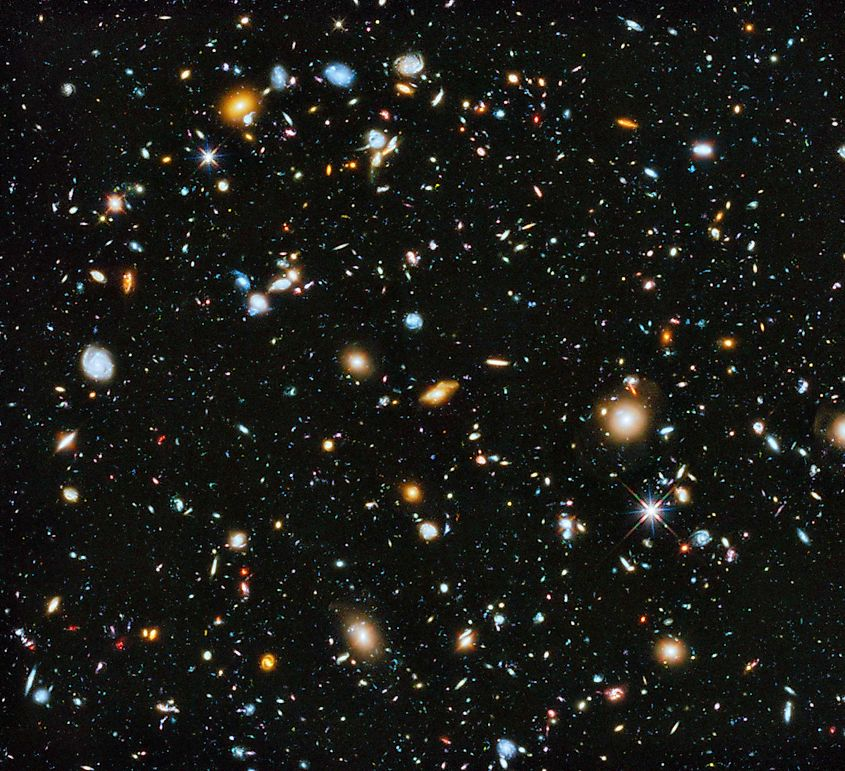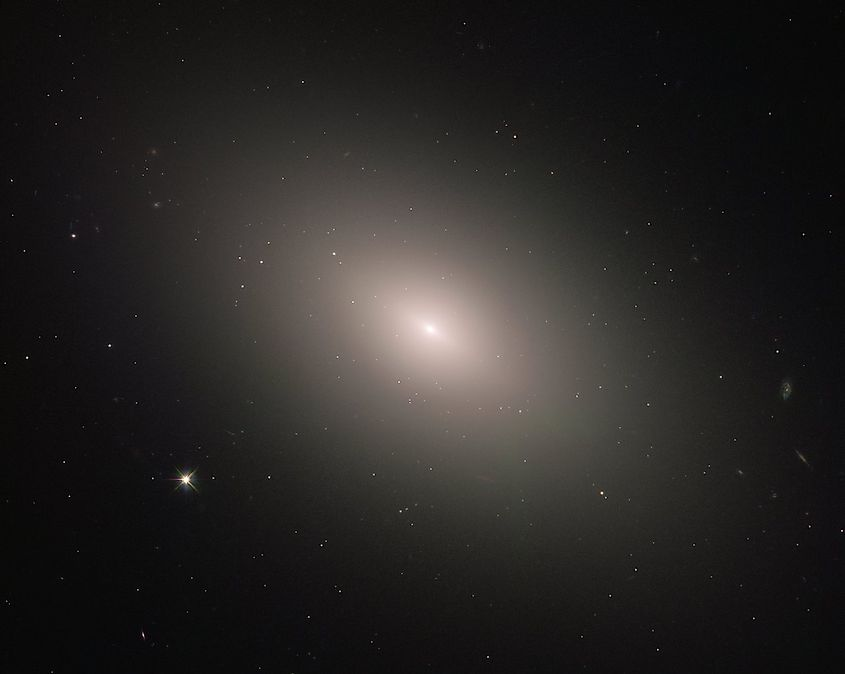# How Fast Is The Universe Expanding?

One of the most fascinating facts about the universe is that space is expanding. The universe is already a gigantic place, and over time, it’s only getting bigger. The expansion of space was first discovered by the astronomer Edwin Hubble in the 1920s. Hubble first discovered that, contrary to what was believed at the time, the universe contained a multitude of galaxies rather than just the Milky Way. While observing distant galaxies, Hubble noticed that most of them appeared red. While the color of distant galaxies may not seem overly significant, the fact that nearly every galaxy appears red suggests that they are all speeding away from us at tremendous speeds. This observation is due to the Doppler Effect, which describes how wavelengths of both light and sound will change depending on an object’s motion. When an object emitting light moves towards us, its light will be compressed and blue-shifted. If that same object is moving away from us, its light will be stretched and red-shifted. While this will be true for any amount of speed, it only becomes noticeable at extreme velocities. Thus, the fact that most galaxies are red-shifted suggests that they are moving away from us at tremendous speeds. Knowing this, just how fast is the universe expanding?

## Calculating the Rate of ExpansionBy knowing the distances to far away galaxies, scientists can calculate the rate of the universe's expansion. Image credit: NASA/ESA

Current estimates on the expansion rate of the universe suggest that space expands at a rate of 73-kilometres per second per megaparsec. Of course, this value does not mean much unless we understand both where it came from and what a megaparsec is. Firstly, a megaparsec is simply a measure of distance used in astronomy. While we generally think of light years when talking about distances in space, the universe itself is so large that light years are not always useful. Rather, astronomers employ the use of parsecs, with one parsec being equal to 3.26-light years. A megaparsec is equal to one-million parsecs or 3.26-million light years. By saying that the universe expands at a rate of 73-kilometres per second per megaparsec, we are saying that, for every one-megaparsec of distance, the universe expands by 73-kilometres. While we now understand what this value means, where did it come from?

The primary method used in calculating the expansion rate of the universe is to calculate how far away distant galaxies are. While this may seem simple enough, calculating distances in space is a rather difficult task. To do so, astronomers must know the brightness of an object since there is a direct relationship between brightness and distance. However, this is where things get difficult. For example, let’s say you have two lightbulbs that both have the same light output. You place one 2-miles away and another 4-miles away. As you might expect, the lightbulb that is further away will appear dimmer than the one that is closer. However, while one appears dimmer than the other, they are both emitting the exact same brightness. The brightness the lightbulbs appear to be is called apparent magnitude, while their actual brightness is called absolute magnitude. Knowing apparent magnitude is rather simple as it is the brightness the object appears to be. Absolute magnitude, however, can be tremendously difficult to calculate. Not every object in space has an absolute magnitude that can be calculated, and so to determine how far away objects are, astronomers look for objects referred to as standard candles. A standard candle is an object whose apparent magnitude changes over short periods of time or whose absolute magnitude can be easily calculated. For example, when Edwin Hubble calculated the distance to other galaxies, he looked for what are known as variable stars. Variable stars are a type of star whose brightness fluctuates over a short period of time, and by knowing the difference between variations in brightness, astronomers can calculate absolute magnitude. While variable stars work well for some galaxies, they are not overly reliable for the most distant galaxies. In more recent years, astronomers have utilized another type of standard candle called Type 1A Supernovae. Type 1A Supernovae are a type of supernovae that occur when white dwarf stars exceed their upper mass limit, causing them to undergo a thermonuclear reaction and explode. Interestingly, since every white dwarf has the same upper mass limit, every Type 1A Supernovae will release the same amount of energy. This allows astronomers to easily calculate their absolute magnitude and thus the distance between us and the supernovae. In the late 1990s, astronomers used Type 1A Supernovae to discover that the expansion of space was accelerating, as well as the overall rate of expansion.

## Other MethodsScientists use the britghness of elliptical galaxies to calculate the distance to them and the rate of expansion. Image credit: NASA/ESA

While the use of Type 1A Supernovae in calculating the expansion rate of the universe works, it is not the only method used. Recently, astronomers have used a different, more accurate method that uses the overall brightness of giant elliptical galaxies. Elliptical galaxies are generally composed almost entirely of colder red stars such as red giants and red dwarfs. By knowing the brightness of these types of stars, astronomers can deduce the absolute magnitude of elliptical galaxies. By knowing brightness, distance can then be calculated, allowing astronomers to calculate the rate at which space expands. Using this method, astronomers have found more evidence that the universe expands at a rate of 73-kilometres per second per megaparsec.